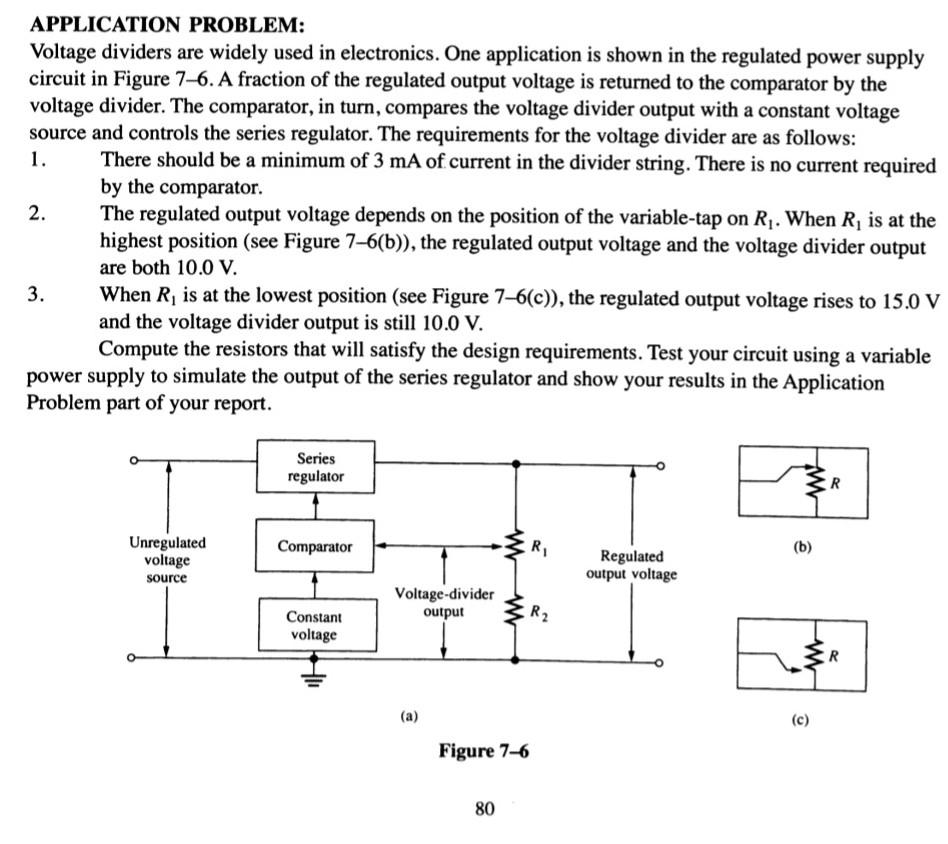# Question APPLICATION PROBLEM: Voltage dividers are widely used in electronics. One application is shown in the regulated power supply circuit in Figure 7-6. A fraction of the regulated output voltage is returned to the comparator by the voltage divider. The comparator, in turn, compares the voltage divider output with a constant voltage source and controls the series APPLICATION PROBLEM: Voltage dividers are widely used in electronics. One application is shown in the regulated power supply circuit in Figure 7-6. A fraction of the regulated output voltage is returned to the comparator by the voltage divider. The comparator, in turn, compares the voltage divider output with a constant voltage source and controls the series regulator. The requirements for the voltage divider are as follows: 1. There should be a minimum of $$3 \mathrm{~mA}$$ of current in the divider string. There is no current required by the comparator. 2. The regulated output voltage depends on the position of the variable-tap on $$R_{1}$$. When $$R_{1}$$ is at the highest position (see Figure 7-6(b)), the regulated output voltage and the voltage divider output are both $$10.0 \mathrm{~V}$$. 3. When $$R_{1}$$ is at the lowest position (see Figure 7-6(c)), the regulated output voltage rises to $$15.0 \mathrm{~V}$$ and the voltage divider output is still $$10.0 \mathrm{~V}$$. Compute the resistors that will satisfy the design requirements. Test your circuit using a variable power supply to simulate the output of the series regulator and show your results in the Application Problem part of your report. (b) (a) (c) Figure 7-6 80JMAEMV The Asker · Electrical EngineeringTranscribed Image Text: APPLICATION PROBLEM: Voltage dividers are widely used in electronics. One application is shown in the regulated power supply circuit in Figure 7-6. A fraction of the regulated output voltage is returned to the comparator by the voltage divider. The comparator, in turn, compares the voltage divider output with a constant voltage source and controls the series regulator. The requirements for the voltage divider are as follows: 1. There should be a minimum of $$3 \mathrm{~mA}$$ of current in the divider string. There is no current required by the comparator. 2. The regulated output voltage depends on the position of the variable-tap on $$R_{1}$$. When $$R_{1}$$ is at the highest position (see Figure 7-6(b)), the regulated output voltage and the voltage divider output are both $$10.0 \mathrm{~V}$$. 3. When $$R_{1}$$ is at the lowest position (see Figure 7-6(c)), the regulated output voltage rises to $$15.0 \mathrm{~V}$$ and the voltage divider output is still $$10.0 \mathrm{~V}$$. Compute the resistors that will satisfy the design requirements. Test your circuit using a variable power supply to simulate the output of the series regulator and show your results in the Application Problem part of your report. (b) (a) (c) Figure 7-6 80
Transcribed Image Text: APPLICATION PROBLEM: Voltage dividers are widely used in electronics. One application is shown in the regulated power supply circuit in Figure 7-6. A fraction of the regulated output voltage is returned to the comparator by the voltage divider. The comparator, in turn, compares the voltage divider output with a constant voltage source and controls the series regulator. The requirements for the voltage divider are as follows: 1. There should be a minimum of $$3 \mathrm{~mA}$$ of current in the divider string. There is no current required by the comparator. 2. The regulated output voltage depends on the position of the variable-tap on $$R_{1}$$. When $$R_{1}$$ is at the highest position (see Figure 7-6(b)), the regulated output voltage and the voltage divider output are both $$10.0 \mathrm{~V}$$. 3. When $$R_{1}$$ is at the lowest position (see Figure 7-6(c)), the regulated output voltage rises to $$15.0 \mathrm{~V}$$ and the voltage divider output is still $$10.0 \mathrm{~V}$$. Compute the resistors that will satisfy the design requirements. Test your circuit using a variable power supply to simulate the output of the series regulator and show your results in the Application Problem part of your report. (b) (a) (c) Figure 7-6 80